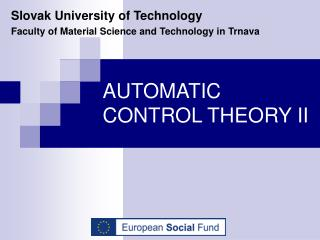DownloadDownload PresentationAUTOMATIC CONTROL THEORY II

# AUTOMATIC CONTROL THEORY II

Download Presentation## AUTOMATIC CONTROL THEORY II

- - - - - - - - - - - - - - - - - - - - - - - - - - - E N D - - - - - - - - - - - - - - - - - - - - - - - - - - -
##### Presentation Transcript

1. Slovak University of Technology Faculty of Material Science and Technology in Trnava AUTOMATIC CONTROL THEORY II

2. Adaptive Control • Model reference adaptive control - MRAC • one of the main approaches to adaptive control • the reference model is chosen to generate the desired trajectory, ym, that the plant output yp has to follow • the tracking error e1 = yp - ym represents the deviation of the plant output from the desired trajectory

3. Adaptive Control • the closed-loop plant is made up of an ordinary feedback control law that contains • contains the plant • a controller C(θ) • an adjustment mechanism that generates the controller parameter estimates θ(t) on-line

4. Adaptive Control • the basic structure of a MRAC scheme

5. Adaptive Control • MRAC schemes can be characterized • as direct • or indirect and • with normalized • or unnormalized adaptive laws

6. Adaptive Control • direct MRAC • the parameter vector θ of the controller C(θ) is updated directly by an adaptive law • indirect MRAC • θ is calculated at each time t by solving a certain algebraic equation that relates θ with the on-line estimates of the plant parameters

7. Adaptive Control • in both direct and indirect MRAC with normalized adaptive laws • the form of C(θ), motivated from the known parameter case, is kept unchanged • the controller C(θ) is combined with • an adaptive law • or an adaptive law and an algebraic equation in the indirect case

8. Adaptive Control • MRAC schemes with unnormalized adaptive laws • C(θ) is modified to lead to an error equation whose form allows the use of the SPR- Lyapunov design approach for generating the adaptive law • the design of C(θ) and adaptive law is more complicated in both the direct and indirect case • the analysis is much simpler and follows from a consideration of a single Lyapunov-like function

9. Adaptive Control • consider the following scalar planta is a constant but unknown • the control objective is • to determine a bounded function u = f(t; x) • such that the state x(t) is bounded • and converges to zero as t → ∞ for any given initial condition x0

10. Adaptive Control • let -am be the desired closed-loop pole where am > 0 is chosen by the designer • Control Law • if the plant parameter a is known • the control lawwithk* = a + am could be used to meet the control objective

11. Adaptive Control • the closed-loop plant iswhose equilibrium xe = 0 is e.s. in the large • because a is unknown • k* cannot be calculated • with k* replaced by its estimate k(t), i.e., we useand search for an adaptive law to update k(t) continuously with time

12. Adaptive Control • Adaptive Law • for generating k(t) is developed by viewing the problem as an on-line identification problem for k* • add and subtract the desired control input -k*x in the plant equation to obtain

13. Adaptive Control • because a – k* = -am we haveor • we can proceed with the SPR-Lyapunov design approach and generate

14. Adaptive Control • the error equationoris in a convenient form for choosing an appropriate Lyapunov function to design the adaptive law for k(t)

15. Adaptive Control • assume that the adaptive law is of the formwhere f1 is some function to be selected, and proposefor some γ > 0 as a potential Lyapunov function for the system

16. Adaptive Control • the time derivative of V along the trajectory ofand is given by

17. Adaptive Control • choosing f1 = γ є1 xwe have